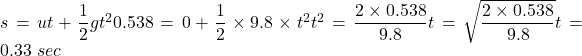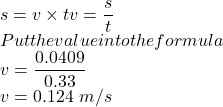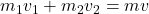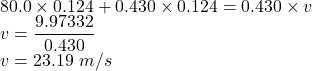## Kyle, a 80.0 kg football player, leaps straight up into the air (with no horizontal velocity) to catch a pass. He catches the 0.430 kg ball

Question

Kyle, a 80.0 kg football player, leaps straight up into the air (with no horizontal velocity) to catch a pass. He catches the 0.430 kg ball precisely at the peak of his jump, when he is 0.538 meters off the ground. He hits the ground 0.0409 meters away from where he leapt. If the ball was moving horizontally when it was caught, how fast was the ball traveling

in progress 0
5 months 2021-08-27T22:34:48+00:00 2 Answers 117 views 0

The velocity of ball is 23.19 m/s

Explanation:

Given that,

Mass of football player = 80.0 kg

Mass of ball = 0.430 kg

Distance = 0.538

We need to calculate the time

Using equation of motionWe need to calculate the velocity

Using formula of velocityWe need to calculate the velocity of ball

Using conservation of momentumPut the value into the formulaThe velocity of ball is 23.19 m/s

23.0m/s

Explanation:

Let the initial velocity of Kyle be u

v² = u² -2gh

Where v = final velocity of Kyle.

h = height above the ground.

g = acceleration due to gravity.

At the highest point v = 0m/s

0 = u² – 2gh

u² = 2gh

Given

h = 0.538m

Taking g = 9.8m/s²

u² = 2×9.8×0.538 = 10.5448

u = 3.25m/s.

Time required to jump that high can be found from the equation

v = u – gt

0 = u – gt

u = gt

t = u/g = 3.25/9.8 = 0.332s

This time is also equal to the time required to get back down from that height to the ground.

Now considering the horizontal motion of kyle,

x = Vx t

Where Vx is the horizontal velocity

Kyle moves a horizontal distance of 0.0409m in t = 0.332s

So Vx = x/t = 0.0409/0.332 = 0.123m/s

His initial horizontal velocity is 0m/s and his final horizontal velocity is 0.123m/s. He share this velocity with the ball he catches in the air as both of them become joined together and move as one body.

The initial momentum of the ball is now shared by Kyle and the ball.

From the principle of conservation of momentum,

P1 + P2 = P

P1 = initial horizontal momentum of kyle

P2 = initial horizontal momentum of the ball

P = final momentum of the ball and Kyle

P1 = 0 (no initial horizontal velocity for kyle)

P2 = mass of ball × initial velocity of ball ub

= 0.430 × ub = 0.43ub

P = (mass of ball + mass of Kyle) × common velocity.

P = (80+0.430) × 0.123 = 9.89kgm/s

Therefore

0 + 0.43ub = 9.89

Ub = 9.89/0.123

Ub = 23m/s.

Considering the horizontal motion of Kyle movi